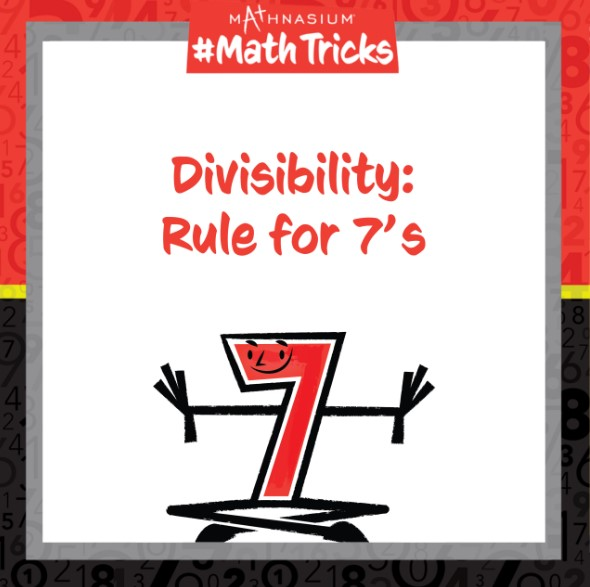877-601-6284
Get Started by Finding a Local Center

# MATHNASIUM #MATHTRICKS: DIVISIBILITY (RULE FOR 7’S)

Mar 28, 2023 | WoodmoreWelcome to Mathnasium’s Math Tricks series. Today we are determining whether a number is divisible by 7.

A number is divisible by 7 if …

5 times the one’s digit PLUS the rest of the digits is divisible by 7.

Follow the examples below to determine whether the numbers are divisible by 7.

##### Example 1: Is 234 divisible by 7?

Step 1: Multiply five times the one’s digit.

5 times the one’s digit, 4, equals 20.

Step 2: Add the rest of the number to the product from Step 1.

The rest of the number, 23, plus the product from Step 1, 20, equals 43.

Step 3: Determine whether the answer from Step 2 is divisible by 7. Repeat Steps 1 and 2, if necessary.

Since 43 is not divisible by 7, 234 is not divisible by 7.

##### Example 2: Is 1,505 divisible by 7?

Step 1: Multiply five times the one’s digit.

5 times the one’s digit, 5, equals 25.

Step 2: Add the rest of the number to the product from Step 1.

The rest of the number, 150, plus the product from Step 1, 25, equals 175.

Step 3: Determine whether the answer from Step 2 is divisible by 7. Repeat Steps 1 and 2, if necessary.

Since it is not easy to determine whether 175 is divisible by 7, we repeat Steps 1 and 2.

5 times the one’s digit, 5, equals 25.

The rest of the number, 17, plus the product 25, equals 42.

Since 42 is divisible by 7, 175 is divisible by 7, and therefore 1,505 is divisible by 7.